## Bonding and Antibonding Molecular Orbitals

Bonding and antibonding orbitals are illustrated in MO diagrams, and are useful for predicting the strength and existence of chemical bonds.

### Learning Objectives

Recognize the relative energies of bonding and antibonding molecular orbitals.

### Key Takeaways

#### Key Points

• The Aufbau principle states that orbitals are filled with the lowest energy first.
• The Pauli exclusion principle states that the maximum number of electrons occupying an orbital is two, with opposite spins.
• Hund’s rule states that when there are several MOs with equal energy, the electrons occupy the MOs one at a time before two occupy the same MO.

#### Key Terms

• antibonding: an atomic or molecular orbital whose energy increases as its constituent atoms move closer together, generating a repulsive force that hinders bonding
• antibonding orbital: one that is located outside the region of two distinct nuclei

### Molecular Orbital Theory

In MO theory, molecular orbitals form by the overlap of atomic orbitals. Atomic orbital energy correlates with electronegativity, as electronegative atoms hold electrons more tightly, lowering their energies. MO modeling is only valid when the atomic orbitals have comparable energy; when the energies differ greatly, the bonding mode becomes ionic. A second condition for overlapping atomic orbitals is that they have identical symmetry.

Two atomic orbitals can overlap in two ways, depending on their phase relationship. An orbital’s phase is a direct consequence of electrons’ wave-like properties. In graphical representations of orbitals, the orbital phase is depicted either by a plus or minus sign (with no relationship to electric charge) or by shading one lobe. The sign of the phase itself does not have physical meaning except when mixing orbitals to form molecular orbitals. Two same-sign orbitals have a constructive overlap, forming a molecular orbital with the bulk of the electron density located between the two nuclei. This MO is called the bonding orbital, and its energy is lower than that of the original atomic orbitals.

### Molecular Orbitals and Symmetry

A bond involving molecular orbitals that are symmetric with respect to rotation around the bond axis is called a sigma bond (σ-bond). If the phase changes, the bond becomes a pi bond (π-bond). Symmetry labels are further defined by whether the orbital maintains its original character after rotating about its center: if so, it is defined gerade, g; if the orbital does not maintain its original character, it is ungerade, u.Hydrogen molecule: Bonding and antibonding levels in the hydrogen molecule; the two electrons in the hydrogen atoms occupy a bonding orbital that is lower in energy than the two separate electrons, making this an energy-favorable event.

Atomic orbitals can also interact with each other out-of-phase, leading to destructive cancellation and no electron density between the two nuclei. In this anti-bonding MO, with energy much higher than the original AOs, any electrons present are located in lobes pointing away from the central internuclear axis. For a corresponding σ-bonding orbital, such an orbital would be symmetrical, but are differentiated from it by an asterisk, as in σ*. For a π-bond, corresponding bonding and antibonding orbitals would not have such symmetry around the bond axis, and are designated π and π* respectively.

### Filling Electrons in MO Diagrams

The next step in constructing an MO diagram is filling the newly formed molecular orbitals with electrons. Three general rules apply:

• The Aufbau principle states that orbitals are filled starting with the lowest energy
• The Pauli exclusion principle states that the maximum number of electrons. occupying an orbital is two, with opposite spins.
• Hund’s rule states that when there are several MOs with equal energy, and the electrons occupy the MOs one at a time before two occupy the same MO.

The filled MO that is highest in energy is called the Highest Occupied Molecular Orbital, or HOMO; the empty MO just above it is the Lowest Unoccupied Molecular Orbital, or LUMO. The electrons in the bonding MOs are called bonding electrons, and any electrons in the antibonding orbital are called antibonding electrons. The reduction these electrons’ energy is the driving force for chemical bond formation.

Whenever symmetry or energy make mixing an atomic orbital impossible, a non-bonding MO is created; often quite similar to and with energy levels equal or close to its constituent AO, the non-bonding MO creates an unfavorable energy event. The resulting electron configuration can be described in terms of bond type, parity, and occupancy; one example is dihydrogen (H2): 1σg2. Sometimes, the letter n designates a non-bonding orbital. The presence of a filled antibonding orbital, after fulfilling the conditions above, indicates that the bond in this case does not exist.The bonding diagram for the hypothetical molecule He2.: Notice the two electrons occupying the antibonding orbital, which explains why the He2 molecule does not exist.

## Bond Order

Bond order is the number of chemical bonds between a pair of atoms.

### Learning Objectives

Calculate a molecule’s bond order given its molecular orbital diagram.

### Key Takeaways

#### Key Points

• Bond order is defined as half the difference between the number of bonding and antibonding electrons.
• Stable bonds have a positive bond order.
• Bond order is an index of bond strength and is used extensively in valence bond theory.

#### Key Terms

• acetylene: ethyne; the simplest alkyne, a hydrocarbon of formula HC≡CH; a colorless gas, with a peculiar, unpleasant odor, formerly used as an illuminating gas but now used in welding and metallurgy
• sigma bond: a covalent atomic bond that is rotationally symmetric about its axis
• bond order: the number of overlapping electron pairs between a pair of atoms
• antibonding: an atomic or molecular orbital whose energy increases as its constituent atoms converge, generating a repulsive force that hinders bonding

Bond order is the number of chemical bonds between a pair of atoms; in diatomic nitrogen (N≡N) for example, the bond order is 3, while in acetylene (H−C≡C−H), the bond order between the two carbon atoms is 3 and the C−H bond order is 1. Bond order indicates the stability of a bond. In a more advanced context, bond order does not need to be an integer.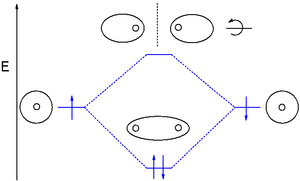Stable dihydrogen molecule: A bond order of one indicates a stable bond.

### Bond Order in Molecular Orbital Theory

In molecular orbital theory, bond order is also defined as the difference, divided by two, between the number of bonding and antibonding electrons; this often, but not always, yields the same result. Bond order is also an index of bond strength, and it is used extensively in valence bond theory.

### Dihydrogen (H2)

This MO diagram depicts the molecule H2, with the contributing AOs on the outside sandwiching the MO. The bonding level (lower level) is completely occupied. A bond order of one is obtained by employing the formula above, indicating a stable bond.

$\text{Bond Order} = \frac{2 (\text{bonding electrons})-0(\text{anti-bonding}\ e-)}{2} = 1$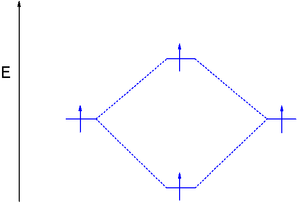Dihydrogen with an electron in the antibonding orbital: By adding energy to an electon and pushing it to the antibonding orbital, this H2 molecule’s bond order is zero, effectively showing a broken bond.

### Dihydrogen (H2) with an Electron in the Antibonding Orbital

In the second diagram, one of the bonding electrons in H2 is “promoted” by adding energy and placing it in the antibonding level.

$\text{Bond} \ \text{Order} = \frac{1 (\text{bonding}\ \text{electrons})-1(\text{anti-bonding}\ \text{e}-)}{2} = 0$

The above formula verifies breaking the H2 bond, which in this case gives a bond order of zero. For a bond to be stable, the bond order must be a positive value.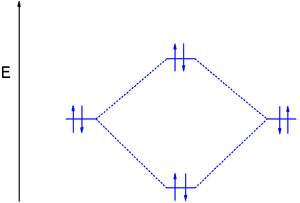The electron configuration of dihelium: If the molecule He2 were to exist, the 4s electrons would have to fully occupy both the bonding and antibonding levels, giving a bond order of zero. Dihelium does not exist.

### Dilithium (Li2)

The last diagram presents the molecule dilithium (Li2). The 1s electrons do not take part in the bonding, but the 2s electrons fill the bonding orbital. The molecule Li2 is a stable molecule in the gas phase, with a bond order of one.

$\text{Bond} \ \text{Order} = \frac{2 (\text{bonding}\ \text{electrons})-0(\text{anti-bonding}\ \text{e}-)}{2} = 1$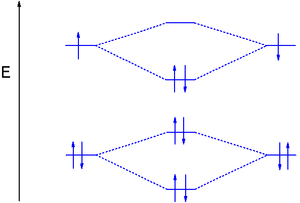The dilithium molecule: Without the 1s electrons participating in bonding, the p electrons completely fill the bonding orbital; this leaves the antibonding orbital empty and gives a bond order of one, indicating a stable molecule (in this case, in the gas phase).

### Dihelium (He2)

The third diagram hypothesizes the molecule dihelium (He2). A bond order of zero is obtained by placing the available electrons in the bonding and antibonding levels, indicating that dihelium does not exist according to valence bond and bond order theory.

$\text{Bond} \ \text{Order} = \frac{2 (\text{bonding}\ \text{electrons})-2(\text{anti-bonding}\ \text{e}-)}{2} = 0$

However, removing an electron from the antibonding level produces the molecule He2+, which is stable in the gas phase with a bond order of 0.5.

$\text{Bond} \ \text{Order} = \frac{2 (\text{bonding}\ \text{electrons})-1(\text{anti-bonding}\ \text{e}-)}{2} = 0.5$

## Linear Combination of Atomic Orbitals (LCAO)

An LCAO approximation is a quantum superposition of atomic orbitals, used to calculate molecular orbitals in quantum chemistry.

### Learning Objectives

Predict which orbitals can mix to form a molecular orbital based on orbital symmetry, and how many molecular orbitals will be produced from the interaction of one or more atomic orbitals

### Key Takeaways

#### Key Points

• Electron configurations of atoms are described as wave functions.
• Wave functions are the basic set of functions that describe a given atom’s electrons.
• In chemical reactions, orbital wave functions are modified—the electron cloud shape is changed—according to the type of atoms participating in the chemical bond.
• The basis functions are one-electron functions centered on the nuclei of component atoms in a molecule.
• Minimizing the total energy of the system determines an appropriate set of linear combinations’ coefficients.
• The shape of the molecular orbitals and their respective energies are approximated by comparing the energies of the individual atoms’ atomic orbitals —or molecular fragments—and applying known values for level repulsion and other similar factors.

#### Key Terms

• wavefunction: a mathematical function describing the propagation of the quantum mechanical wave associated with a particle (or system of particles), related to the probability of finding the particle in a particular region of space
• orbital: a specification of an electron’s energy and probability density at any point in an atom or molecule
• diatomic: consisting of two atoms

An atomic orbital is a mathematical function that describes the wave-like behavior of either one electron or a pair of electrons in an atom. This function can be used to calculate the probability of finding any electron in any specific region around an atom’s nucleus. An orbital may also refer to the physical region where the electron can be calculated to exist, as defined by the orbital’s particular mathematical form.

### Describing Molecules

Molecules are built from two or more bound atoms. It is possible to combine the known orbitals of constituent atoms in a molecule to describe its electron orbitals. Molecular orbitals (MOs) represent regions in a molecule where an electron is likely to be found; they are obtained by combining atomic orbitals. An MO can specify a molecule’s electron configuration, and most commonly, it is represented as a linear combination of atomic orbitals (the LCAO-MO method), especially in qualitative or approximate usage. These models provide a simple model of molecule bonding, understood through molecular orbital theory.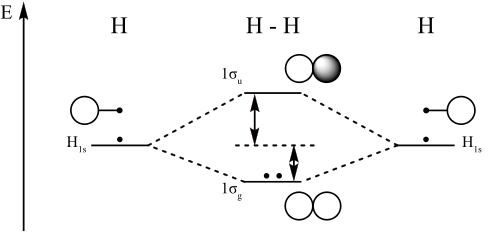Molecular orbital diagram for hydrogen: For a diatomic molecule, an MO diagram effectively shows the energetics of the bond between the two atoms, whose AO unbonded energies are shown on the sides. The unbonded energy levels are higher than those of the bound molecule, which is the energetically-favored configuration.

### Linear Combination of Atomic Orbitals

A linear combination of atomic orbitals, or LCAO, is a quantum superposition of atomic orbitals and a technique for calculating molecular orbitals in quantum chemistry. In quantum mechanics, electron configurations of atoms are described as wave functions. In a mathematical sense, these wave functions are the basic functions that describe the a given atom’s electrons. Orbital wave functions are modified in chemical reactions—the electron cloud shape changes—according to the type of atoms participating in the chemical bond.

One of LCAO’s initial assumptions is that the number of molecular orbitals is equal to the number of atomic orbitals included in the linear expansion. Essentially, n atomic orbitals combine to form n molecular orbitals.

Molecular orbital diagrams are diagrams of MO energy levels, shown as short horizontal lines in the center. Atomic orbitals (AO) energy levels are shown for comparison. Lines, often dashed diagonal lines, connect MO levels with their constituent AO levels. Levels with the same energy are commonly shown side by side. Appropriate AO and MO levels are filled with electrons symbolized by small vertical arrows, whose directions indicate the electron spins.

## Homonuclear Diatomic Molecules

Homonuclear diatomic molecules are composed of only one element.

### Learning Objectives

Recognize the properties of homonuclear diatomic molecules.

### Key Takeaways

#### Key Points

• Diatomic molecules are always linear.
• Diatomic molecules have quantized energy levels for rotation and vibration.
• The halogen series contains many homonuclear diatomic molecules.
• Hydrogen, nitrogen, and oxygen are stable homonuclear diatomic molecules.

#### Key Terms

• homonuclear: having atoms of only one element, especially elements of only a single isotope
• diatomic: consisting of two atoms

Diatomic molecules are composed of only two atoms, of either the same or different chemical elements. Common diatomic molecules include hydrogen (H2), nitrogen (N2), oxygen (O2), and carbon monoxide (CO). Seven elements exist as homonuclear diatomic molecules at room temperature: H2, N2, O2, F2, Cl2, Br2, and I2. The bond in a homonuclear diatomic molecule is non-polar due to the electronegativity difference of zero.

### Geometry

All diatomic molecules are linear, which is the simplest spatial arrangement of atoms.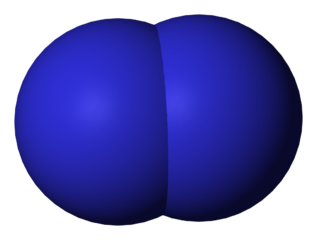Nitrogen: A space-filling model of the homonuclear diatomic molecule nitrogen. Note the inevitable linear geometry.

### Energy Levels

It is convenient and common to represent a diatomic molecule as two point masses (the two atoms) connected by a massless spring. The energies involved in the molecule’s various motions can then be broken down into three categories:

• Translational energies (the molecule moving from point A to point B)
• Rotational energies (the molecule spinning about its axis)
• Vibrational energies (the molecules vibrating in a variety of ways)

## Heteronuclear Diatomic Molecules

Heteronuclear diatomic molecules are composed of two atoms of two different elements.

### Learning Objectives

Recognize when the atomic orbitals in a heteronuclear diatomic molecule will mix.

### Key Takeaways

#### Key Points

• In heteronuclear diatomic molecules, atomic orbitals only mix when the electronegativity values are similar.
• While MOs for homonuclear diatomic molecules contain equal contributions from each interacting atomic orbital, MOs for heteronuclear diatomics contain different atomic orbital contributions.
• Orbital interactions to produce bonding or antibonding orbitals in heteronuclear diatomics occur if there is sufficient overlap between atomic orbitals, as determined by their symmetries and similarity in orbital energies.

#### Key Terms

• diatomic: consisting of two atoms
• heteronuclear: having different types of atoms or nuclei

In heteronuclear diatomic molecules, atomic orbitals only mix when the electronegativity values are similar. In carbon monoxide (CO), the oxygen 2s orbital is much lower in energy than the carbon 2s orbital, so the degree of mixing is low. The g and u subscripts no longer apply because the molecule lacks a center of symmetry.

In hydrogen fluoride (HF), the hydrogen 1s orbital can mix with the fluorine 2pz orbital to form a sigma bond because experimentally, the energy of 1s of hydrogen is comparable with 2p of fluorine. The HF electron configuration reflects that the other electrons remain in three lone pairs and that the bond order is one.

While MOs for homonuclear diatomic molecules contain equal contributions from each interacting atomic orbital, MOs for heteronuclear diatomics contain different atomic orbital contributions. Orbital interactions that produce bonding or antibonding orbitals in heteronuclear diatomics occur if there is sufficient overlap between atomic orbitals, as determined by their symmetries and similarity in orbital energies.

### Examples of Heteronuclear Diatomic Molecules

In hydrogen fluoride, HF, symmetry allows for overlap between the H 1s and F 2s orbitals, but the difference in energy between the two atomic orbitals prevents them from interacting to create a molecular orbital. Symmetry also allows for overlap between the H 1s and F 2pz orbitals, and these two atomic orbitals have a small energy separation; they therefore interact, creating σ and σ* MOs and a molecule with a bond order of one.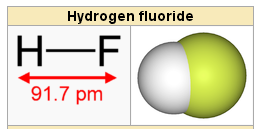Hydrogen fluoride: The hydrogen fluoride molecule.

Hydrogen chloride, HCl, is a diatomic molecule consisting of a hydrogen atom H and a chlorine atom Cl connected by a covalent single bond. Since the chlorine atom is much more electronegative than the hydrogen atom, the covalent bond between the two atoms is quite polar. Consequently, the molecule has a large dipole moment with a negative partial charge δ- at the chlorine atom and a positive partial charge δ+ at the hydrogen atom. In part because of its high polarity, HCl is very soluble in water (and in other polar solvents).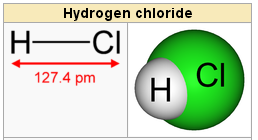Hydrogen chloride: Hydrogen chloride is a diatomic molecule.

Carbon monoxide, CO, has a total of 10 valence electrons. To satisfy the octet rule for the carbon, the two atoms form a triple bond with six shared electrons in three bonding molecular orbitals. Since four of the shared electrons come from the oxygen atom and only two from carbon, one of the bonding orbitals is occupied by two electrons from oxygen.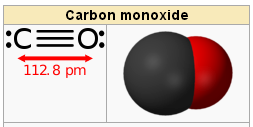Carbon monoxide: Carbon monoxide.

Chlorine monofluoride can convert metals and non-metals to their fluorides, releasing Cl2 in the process; it converts tungsten to tungsten hexafluoride and selenium to selenium tetrafluoride, for example. ClF is a colorless gas at room temperature and is stable even at high temperatures. When cooled to −100 °C, ClF condenses as a pale yellow liquid. Many of its properties are intermediate between its parent halogens, Cl2 and F2.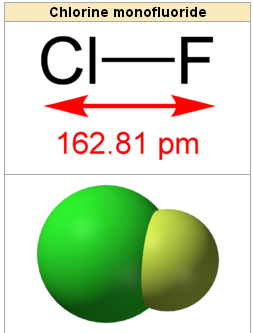Chlorine monofluoride: The interhalogen molecule, chlorine monofluoride.

### Heteronuclear Diatomic Molecules and Their Dipole Moments

To determine exact polarity, dipole moment (in Debye ) can be calculated as the product of the separated charges (Q) and distance between them (r) in Angstroms:

$\mu=\text{Qr}$

Finding the value of Q can be challenging, but the value is easily converted from the percent ionic character of a bond—simply convert the percent to decimal by dividing by 100; r is simply the bond length.

Sample problem: What is the dipole moment of the Cl-F molecule with a bond length of 163 picometers (163 x 10-12 m) and an 11 percent ionic character? (1D = 3.36 x 10-30 Cm)(1e = 1.60 x 10-19 C)

$\mu= (1 \times1.60 \times 10^{-19}\text{C}) \times 163 \times 10^{-12}\text{m}$

$\mu= 2.61 \times 10^{-29}\text{Cm}$

Solve for the value in Debye (this value represents the molecule with 100 percent ionic character):

$\text{D} = \frac{2.61\times10^{-29}}{3.36\times10^{-30}} = 7.8 \text{D}$

For 11 percent ionic character:

D = 7.8 x.11 =.86 D

## Polyatomic Molecules

A polyatomic molecule is a single entity composed of at least three covalently-bonded atoms.

### Learning Objectives

Recognize the properties of a polyatomic molecule.

### Key Takeaways

#### Key Points

• Polyatomic molecules consist of a stable system (bound state) comprising three or more atoms.
• The molecular formula characterizes different molecules by reflecting their exact number of compositional atoms.
• The empirical formula is often, but not always, the same as the molecular formula.

#### Key Terms

• empirical formula: a notation indicating the ratios of the various elements present in a compound, without regard to the actual numbers

Polyatomic molecules are electrically neutral groups of three or more atoms held together by covalent bonds. Molecules are distinguished from ions by their lack of electrical charge.

### Molecular Chemistry and Molecular Physics

The science of molecules is called molecular chemistry or molecular physics, depending on the focus. Molecular chemistry deals with the laws governing the interaction between molecules resulting in the formation and breakage of chemical bonds; molecular physics deals with the laws governing their structure and properties. In molecular sciences, a molecule consists of a stable system (bound state) comprising two or more atoms. Molecules have fixed equilibrium geometries—bond lengths and angles—about which they continuously oscillate through vibrational and rotational motions.

A pure substance is composed of molecules with the same average geometrical structure. A molecule’s chemical formula and structure are the two important factors that determine its properties, particularly reactivity.

A compound ‘s empirical formula is the simplest integer ratio of its constitutional chemical elements. For example, water is always composed of a 2:1 ratio of hydrogen to oxygen atoms.Water: Another triatomic composed of two atoms, hydrogens (white) are bound to a central oxygen (red); note that this molecule is not linear.

Ethyl alcohol, or ethanol, is always composed of carbon, hydrogen, and oxygen in a 2:6:1 ratio; this does not uniquely determine the kind of molecule, however. Dimethyl ether, for example, has the same ratios as ethanol. Molecules with the same atoms in different arrangements are called isomers. For example, carbohydrates have the same ratio (carbon: hydrogen: oxygen = 1:2:1) and thus the same empirical formula, but have different total numbers of atoms in the molecule.

### Molecular and Empirical Formulas

The molecular formula characterizes different molecules by reflecting their exact number of compositional atoms. Different isomers can have the same atomic composition while being different molecules, however. The empirical formula is often the same as the molecular formula, but not always; for example, the molecule acetylene has molecular formula C2H2, but the simplest integer ratio of elements is CH.